# Online Verbal Reasoning Test - Verbal Reasoning Test 10Loading Test...

Instruction:

• This is a FREE online test. DO NOT pay money to anyone to attend this test.
• Total number of questions : 20.
• Time alloted : 30 minutes.
• Each question carry 1 mark, no negative marks.
• DO NOT refresh the page.
• All the best :-).

1.

A child went 90 m in the East to look for his father, then he turned right and went 20 m. After this he turned right and after going 30 m he reached to his uncle's house. His father was not there. From there he went 100 m to his north and met his father. How far did he meet his father from the starting point?

A.
 80 mB.
 100 mC.
 140 mD.
 260 mExplanation: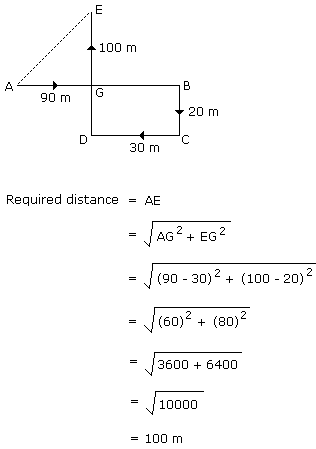2.

If A + B means A is the brother of B; A x B means A is the son of B; and A % B means B is the daughter of A then which of the following means M is the maternal uncle of N?

A.
 M + O x NB.
 M % O x N + PC.
 M + O % ND.
 None of theseExplanation:

Because the sex of O is not known.

3.

If A + B means B is the brother of A; A x B means B is the husband of A; A - B means A is the mother of B and A % B means A is the father of B, which of the following relations shows that Q is the grandmother of T?

A.
 Q - P + R % TB.
 P x Q % R - TC.
 P x Q % R + TD.
 P + Q % R - TExplanation:

Q - P → Q is the mother of P

P + R → R is the brother of P

Hence, → q is the mother of R

R % T → R is the father of T.

Hence, Q is the grandmother of T.

4.

If A \$ B means A is the brother of B; B * C means B is the son of C; C @ D means C is the wife of D and A # D means A is the son of D, how C is related to A?

A.
 Maternal grandmotherB.
 Maternal auntC.
 AuntD.
 MotherExplanation:

A \$ B → A is the brother of B

B * C → B is the son of C

Hence, → A is the son of C

C @ D → C is the wife of D

Hence, → C is the mother of A.

Direction (for Q.Nos. 5 - 6):

In each of the following questions two statements are given and these statements are followed by two conclusions numbered (1) and (2). You have to take the given two statements to be true even if they seem to be at variance from commonly known facts. Read the conclusions and then decide which of the given conclusions logically follows from the two given statements, disregarding commonly known facts.

• (A) If only (1) conclusion follows
• (B) If only (2) conclusion follows
• (C) If either (1) or (2) follows
• (D) If neither (1) nor (2) follows and
• (E) If both (1) and (2) follow.
5.

Statements: Some papers are pens. All the pencils are pens.

Conclusions:

1. Some pens are pencils.
2. Some pens are papers.

A.
 Only (1) conclusion followsB.
 Only (2) conclusion followsC.
 Either (1) or (2) followsD.
 Neither (1) nor (2) followsE.
 Both (1) and (2) followExplanation: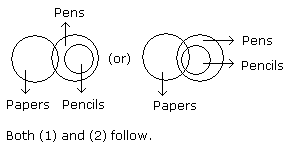6.

Statements: All cars are cats. All fans are cats.

Conclusions:

1. All cars are fans.
2. Some fans are cars.

A.
 Only (1) conclusion followsB.
 Only (2) conclusion followsC.
 Either (1) or (2) followsD.
 Neither (1) nor (2) followsE.
 Both (1) and (2) followExplanation: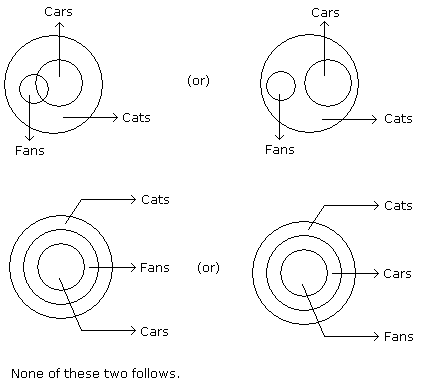Direction (for Q.No. 7):
In each of the following questions there are three statements. Which are followed by three or four conclusions. Choose the conclusions which logically follow from the given statements.
7.

Statements: All the papers are books. All the bags are books. Some purses are bags.

Conclusions:

1. Some papers are bags.
2. Some books are papers.
3. Some books are purses.

A.
 Only (1)B.
 Only (2) and (3)C.
 Only (1) and (2)D.
 Only (1) and (3)Explanation: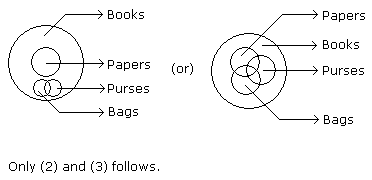8.

Yesterday I saw an ice cube which had already melted due to heat of a nearby furnace.

A.
 AlwaysB.
 NeverC.
 OftenD.
 SometimesExplanation:

Since the ice cube had already melted due to the heat of a nearby furnace so after this ice cannot remain as ice cube.

9.

Two positions of a dice are shown below. When number '1' is on the top. What number will be at the bottom?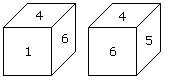A.
 3B.
 5C.
 2D.
 6Explanation:

According to the rule (2) when 'one' is at the top, then 5 will be at the bottom.

10.

Here 4 positions of a cube are shown. Which sign will be opposite to '+' ?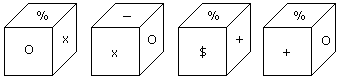A.
 %B.
 -C.
 xD.
 \$Explanation:

From position I and III common face with % is in the same position. Hence according to rule (3) opposite is X.

Direction (for Q.No. 11):
The figure given on the left hand side in each of the following questions is folded to form a box. Choose from the alternatives (1), (2), (3) and (4) the boxes that is similar to the box formed.
11.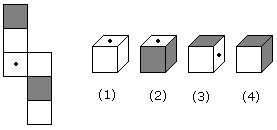A.
 2 and 3 onlyB.
 1, 3 and 4 onlyC.
 2 and 4 onlyD.
 1 and 4 onlyDirection (for Q.No. 12):

The following questions are based on the information given below:

1. There is a cuboid whose dimensions are 4 x 3 x 3 cm.
2. The opposite faces of dimensions 4 x 3 are coloured yellow.
3. The opposite faces of other dimensions 4 x 3 are coloured red.
4. The opposite faces of dimensions 3 x 3 are coloured green.
5. Now the cuboid is cut into small cubes of side 1 cm.
12.

How many small cubes will have only one face coloured ?

A.
 10B.
 12C.
 14D.
 18Explanation: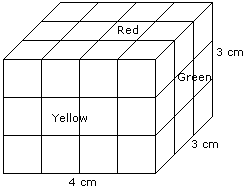Number of small cubes having only one face coloured = 2 x 2 + 2 x 2 + 2 x 1

= 4 + 4 + 2

= 10

Direction (for Q.No. 13):
A cube is cut in two equal parts along a plane parallel to one of its faces. One piece is then coloured red on the two larger faces and green on the remaining, while the other is coloured green on two smaller adjacent faces and red on the remaining. Each is then cut into 32 cubes of same size and mixed up.
13.

How many cubes have no coloured face at all ?

A.
 32B.
 8C.
 16D.
 NoneExplanation: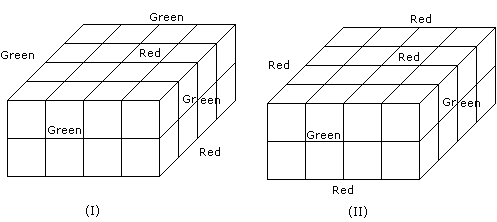There is no such cube in all, where all the faces are unclosured.

Direction (for Q.No. 14):
In each of the following questions find out the alternative which will replace the question mark.
14.

DFHJ : LNPR :: TVXZ : ?

A.
 DBFHB.
 DBHFC.
 BDFHD.
 FDBHExplanation: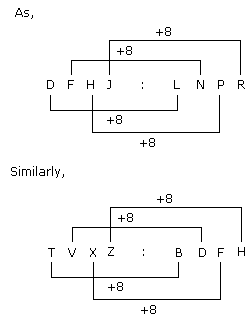Direction (for Q.No. 15):
P, Q, R, S, T, U, V and W are sitting round the circle and are facing the centre:
1. P is second to the right of T who is the neighbour of R and V.
2. S is not the neighbour of P.
3. V is the neighbour of U.
4. Q is not between S and W. W is not between U and S.
15.

Which two of the following are not neighbours ?

A.
 RVB.
 UVC.
 RPD.
 QWExplanation: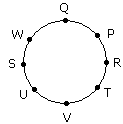16.

Which one will replace the question mark ?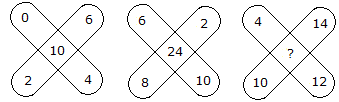A.
 36B.
 48C.
 38D.
 30Explanation:

(0 + 2 + 6 + 4) - 2 = 10

and (6 + 2 + 10 + 8) - 2 = 24

Therefore, (4 + 14 + 12 + 10) - 2 = 38.

17.

Which one will replace the question mark ?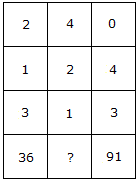A.
 25B.
 59C.
 48D.
 73Explanation:

(2)3 + (1)3 + (3)3 = 36

and (0)3 + (4)3 + (3)3 = 91

Therefore, (4)3 + (2)3 + (1)3 = 73.

18.

Which one will replace the question mark ?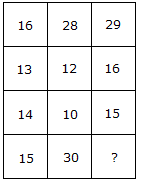A.
 60B.
 30C.
 20D.
 45Explanation:

(16 + 13) = (14 + 15)

and (28 + 12) = (10 + 30)

Therefore (29 + 16) = (15 + 30).

19.

Which one will replace the question mark ?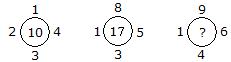A.
 18B.
 20C.
 21D.
 19Explanation:

1 + 2 + 3 + 4 = 10

and 1 + 3 + 5 + 8 = 17

Similarly, 1 + 4 + 6 + 9 = 20.

20.

Which one will replace the question mark ?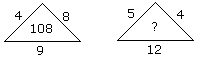A.
 80B.
 114C.
 108D.
 None of theseExplanation:

(4 + 8) x 9 = 108

(5 + 4) x 12 = 108.